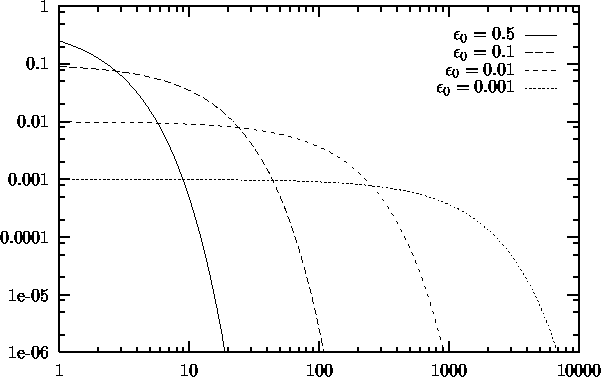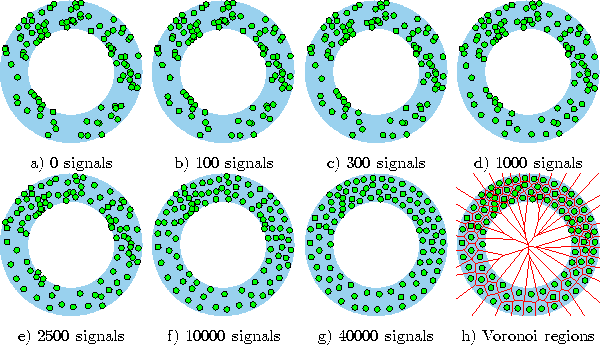Next: k-means Up: Hard Competitive Learning Previous: On-line Update: Basic Algorithm

# Constant Learning Rate

If the learning rate is constant, i.e.then the value of each reference vectorrepresents an exponentially decaying average of those input signals for which the unit c has been winner. To see this, letbe the sequence of input signals for which c is the winner. The sequence of successive values taken bycan then be written asFrom (4.8) and (4.11) it is obvious that the influence of past input signals decays exponentially fast with the number of further input signals for which c is winner (see also figure 4.2). The most recent input signal, however, always determines a fractionof the current value of. This has two consequences. First, such a system stays adaptive and is therefore in principle able to follow also non-stationary signal distribution. Second (and for the same reason), there is no convergence. Even after a large number of input signals the current input signal can cause a considerable change of the reference vector of the winner. A typical behavior of such a system in case of a stationary signal distribution is the following: the reference vectors drift from their initial positions to quasi-stationary positions where they start to wander around a dynamic equilibrium. Better quasi-stationary positions in terms of mean square error are achieved with smaller learning rates. In this case, however, the system also needs more adaptation steps to reach the quasi-stationary positions.Figure 4.2: Influence of an input signalon the vector of its winner s as a function of the number of following input signals for which s is winner (including). Results for different constant adaptation rates are shown. The respective section with the x-axis indicates how many signals are needed until the influence ofis below. For example if the learning rateis set to 0.5, about 10 additional signals (the section with the x-axis is near 11) are needed to let this happen.

If the distribution is non-stationary then the information about the non-stationarity (how rapidly does the distribution change) can be used to set an appropriate learning rate. For rapidly changing distributions relatively large learning rates should be used and vice versa. Figure 4.3 shows some stages of a simulation for a simple ring-shaped data distribution. Figure 4.4 displays the final results after 40000 adaptation steps for three other distribution. In both cases a constant learning ratewas used.Figure 4.3:   Hard competitive learning simulation sequence for a ring-shaped uniform probability distribution. A constant adaptation rate was used. a) Initial state. b-f) Intermediate states. g) Final state. h) Voronoi tessellation corresponding to the final state.Figure 4.4:   Hard competitive learning simulation results after 40000 input signals for three different probability distributions. A constant learning rate was used. a) This distribution is uniform within both shaded areas. The probability density, however, in the upper shaded area is 10 times as high as in the lower one. b) The distribution is uniform in the shaded area. c) In this distribution each of the 11 circles indicates the standard deviation of a Gaussian kernel which was used to generate the data. All Gaussian kernels have the same a priori probability.Next: k-means Up: Hard Competitive Learning Previous: On-line Update: Basic Algorithm

Bernd Fritzke
Sat Apr 5 18:17:58 MET DST 1997